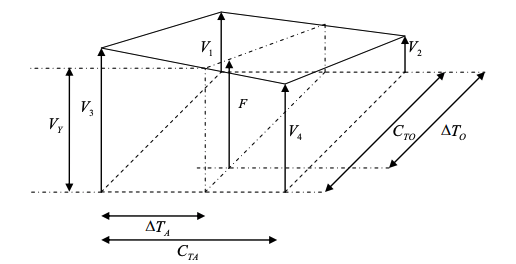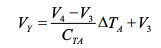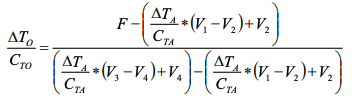# Calculation Of Temperature

Calculation Of The Object Temperature Derived From 4 Sampling Points Using Linear InterpolationSelection of 4 sampling points (sensor voltages) V1 ,V2 ,V3 and V4
can be done according to following
principle :
Measurement of the thermopile voltage and temperature reference signal. Determination of the actual
sensor temperature derived from the measured temperature reference signal.
The calculated sensor
temperature is used to find the bordering ambient temperature rows in the data array. Now the sampling points for the interpolation can be defined, if one of the array data isn’t the value looked for accidentally.
The values of the selected rows are searched for the largest value less than the measured thermopile
voltage. The search starts at the lowest object temperature row in the two selected ambient temperature rows.
The following equation helps to find the right points e.g. by a software program. The right sampling points have been selected, if the condition “ VY> F” is complied. „F“ stands for the measured thermopile voltage.V1 ,V2 ,V3 ,V4 -> Sampling points from the data array
F -> Thermopile voltage
CTO -> Step range of the object temperature axis (temperature difference between 2 rows)
CTA -> Step range of the ambient temperature axis
TA -> Difference between sensor temperature and axis temperature related to the sampling points
TO -> Difference between calculated object temperature and axis temperature related to the sampling points.

The object temperature can be calculated by linear interpolation using following equation